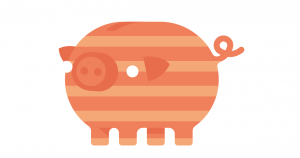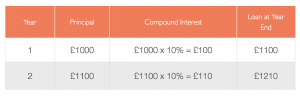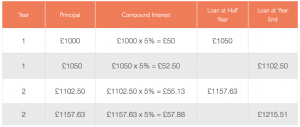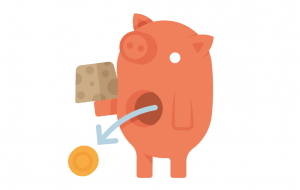# How Much Interest do You Pay or Earn?

The amount of interest you pay or earn depends on several factors:

Original amount borrowed for a debt, or deposited in a bank or savings account (the ‘principal’)
Length of time you borrow or save (the ‘term’)
Interest rate %, and whether that rate is variable or fixed
Method used to calculate interest (simple or compound)
Frequency that interest is added (e.g. daily, monthly, quarterly, annually)

As well as interest, the borrower and lender also agree on a repayment schedule, and penalties if the borrower fails to keep to the plan. The contract may also include additional charges such as administration fees.

Let’s look at how each of the above factors affect the amount of interest you pay or earn.### Original Amount – the Principal

The higher the sum originally borrowed or deposited, the more interest you will pay or earn.

### Length of Time – the Term

The longer the period you borrow or save, the longer your debt or savings accumulates interest, therefore the higher the amount of interest added.

The length of the term can have a big impact on the overall interest payment.

### Interest Rate %

Interest rate is the fee charged for borrowing, usually expressed as an annual percentage of the principal amount borrowed.

Example:

If you took out a one year loan of £1000, at an interest rate of 5% per year, you would pay back a £50 interest charge, in addition to the original £1000 you borrowed.

#### Standardised Interest Rates:  APR & AER

When assessing borrowing or savings options, you need to be sure you are comparing like with like. Providers quote various types of interest rates, terms and charges which can get confusing!  So a standardised interest rate was introduced, to give customers a fair and easy way to compare products and providers:

for borrowing

APR shows the total amount a debt would cost if you borrowed the money for one year

for savings and bank accounts in credit

AER shows how much interest would be earned if money was left in an account for one year

### Interest Rate: Variable or Fixed

Borrowing and savings offer a choice between variable or fixed rates, so it’s important to understand the difference, and their impact on interest payments.

#### Variable RateThe interest rate applied to the balance of your debt/savings fluctuates up and down in line with the Bank of England base rate or competitive market forces.  As rates fluctuate, so do interest payments.

• For borrowing, you would benefit from any drop in interest rates, which will be reflected in reduced monthly repayments.  But if interest rates go up, so do monthly repayments and the overall cost of the debt!
• For savings, a rise or fall in interest rate will affect any regular income you receive, as well as the overall value of your investment.

#### Fixed RateThe interest rate remains fixed throughout the term, no matter what happens to market interest rates. The borrower pays back a fixed percentage of the principal amount borrowed, and repayments are the same over the whole term.

Deciding between a fixed or variable rate depends on a number of factors, including your financial situation, the market environment and the duration of the debt or savings account.

#### Find out more:

Borrowing – Certainty v Flexibility: Fixed or variable interest rates

Choosing Savings Accounts: Fixed or Variable Rate?

### Calculation Method: Simple or Compound

The difference between the two methods of calculating interest can have a big impact on your debts or savings, so it’s useful to have a basic understanding.

#### Simple Interest

Simple interest is occasionally used for short-term loans; interest rates tend to be high.

Simple or ‘flat rate’ interest is calculated as a fixed percentage of the amount borrowed at the start (the  principal). The amount of interest charged is always the same, every time it is calculated.

Example: Simple Interest Loan

£1000 loan at 10% simple interest, borrowed for 3 years:

Annual simple interest payment is 10% of the £1000 loan
i.e. £100 per year, for every year of the loan
So at the end of Year 3, accrued interest is 3 x £100
Total amount to be repaid is £1300 (£1000 principal loan + £300 simple interest)

Formula for calculation of Simple Interest:

I   =   P   R   T
Interest = Principal × Rate × Time

I    Interest
P   Principal: the amount borrowed/lent at the start
R   Rate of interest per year (fixed % of the principal)
T    Time: number of years

For the example above:

P          R        T   =     I
1000  x   0.1   x  3   =   300

#### Compound InterestCompound interest is a bit more complicated, but far more common because it earns more for the lender.
Interest payments are higher and money grows more quickly, because as well as earning interest on the original amount, the lender also earns interest on the interest.

Compound interest is calculated as a percentage of the principal amount PLUS accumulated interest. This simply means that at the end of a compounding period, interest is added to the principal amount. Then the next period, the interest that has been added also earns interest… and so on.

Example: Compound Interest Loan

£1000 loan at 10% compound interest, borrowed for 3 years:

10% interest is calculated on the new higher balance at the end of each year.  So the interest payment will increase every year of the loan.
At the end of Year 3, total amount to be repaid is £1331 (£1000 principal loan + £331 compound interest)

The amount of interest increases every time it is calculated, which means your debt or savings grow faster and faster the longer you leave them unchanged.

Compound interest is great for savers …

If you leave your savings in your account without making withdrawals, you will maximise your earnings.

…but it can be bad news for borrowers!

Interest rates are usually higher for borrowing, therefore the compounding effect is even more marked; so if you make only minimum repayments, the amount owed will quickly increase – which is why a small loan can soon spiral into a large debt!### Frequency of Adding or Compounding Interest

Interest may be added or compounded annually, quarterly, monthly, weekly or even daily; the more frequent the compounding periods, the faster interest accrues, so the faster the debt or savings will grow.

A higher interest rate may generate less interest than a lower rate compounded more frequently over the same time period.

Example:  Compare loans A and B at the year ends

#### Loan A:  10% interest rate compounded annually#### Loan B:  5% interest rate compounded twice a year#### Find out more about Interest Rates:

Annual Percentage Rate APR

Annual Equivalent Rate AER

Interest Rates Guide – Money Saving Expert#### The cost of a debt will increase faster:

If interest is calculated using the compounding method

The higher the % interest rate (APR)

The more frequently interest is added or compounded

The longer it remains unpaid#### Savings will grow faster:

If interest is calculated using the compounding method

The higher the % interest rate (AER)

The more frequently interest is added or compounded

The longer savings remain untouched (no withdrawals)

### Tax on Interest Earned – Gross or Net InterestSavings interest counts as income, and is therefore included in Income Tax calculations; however since the introduction of the tax-free Personal Savings Allowance (PSA) in 2016, most people won’t need to pay any tax on their savings.

For any savings income above your allowance, tax will be deducted from the gross interest paid to you by banks and building societies – the remaining amount you receive is net interest.

 Gross Interest Banks & building societies pay gross interest into your account (with no tax taken off the amount) All savings income up to your Personal Savings Allowance limit is tax-free (i.e. gross interest) ↓ LESS Tax & any Charges You pay income tax only on savings interest over your tax-free Allowance ↓ Net Interest This is the interest you actually receive on any savings income above your Allowance, once tax has been deducted

Example: Gross v Net Interest

For a basic rate taxpayer, who will be taxed on savings income above their Personal Savings Allowance, this example shows the effects of tax deduction on gross earnings and interest rate.

£2000 savings over the Allowance limit, in an account offering 5% interest per year:

Gross interest earned = £100   (2000 x 0.05 = 100)

Gross interest is taxed at 20% basic rate = £20 tax deducted

So actual earnings = £80 net interest, which equates to a net interest rate of  80/2000 = 4%

Tip:

For savings which will be taxed…

To calculate net earnings, just multiply the gross by 0.8

(Basic rate tax is 20% = 0.2, so net earnings are 80% = 0.8)

#### Find out more:

Tax on Savings

Tax on Savings Interest – GOV.UK